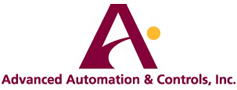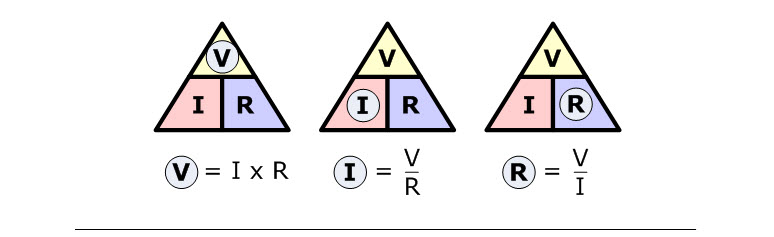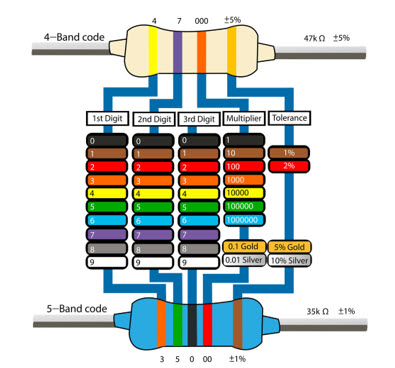# Tech Terms

## OHMS Law

### To find the Voltage, ( V ):

[ V = I x R ] V (volts) = I (amps) x R (Ω)

### To find the Current, ( I ):

[ I = V ÷ R ] I (amps) = V (volts) ÷ R (Ω)

### To find the Resistance, ( R ):

[ R = V ÷ I ] R (Ω) = V (volts) ÷ I (amps)## Horse Power Calculations:

To find the electric horsepower of a motor, use the equation:

### Direct Current

Horsepower = (Volts x Amps) * Efficiency/ 746

### Single Phase

Horsepower = (Volts x Amps) * Efficiency*Power Factor*1.73/ 746

### Three Phase

Horsepower = (Volts x Amps) * Efficiency*Power Factor/ 746

## Calculate Power (Watts)

Power (Watts) = Voltage (E) * Current (I)

### Direct Current:

KiloWatts (KW) = Voltage (E) * Current (I) / 1000

### Single Phase:

KW = E * I * Power Factor (PF) / 1000

### Three Phase:

KW = E * I * PF * 1.73 / 1000

### Resistor Table (How to identify the size of a resistor):## Common Industry Acronyms:

AC = Alternating Current
AGA = American Gas Association
AO = Analog Output
AWG = American Wire Gauge
BIOS = Basic Input/Output System
BPS = Bits Per Second
C/S = Client/Server
CIU = Computer Interface Unit
CNG = Compressed Natural Gas
CPU = Central Processing Unit
CRC = Cyclic Redundancy Checking
CU = Control Unit
DAS = Data Acquisition System
dB =Decibel
DC = Direct Current
DCS = Distributed Control System
DH = Data HI way
DI = Digital Input
DO = Digital Output
DOS = Disk Operating System
DP = Differential Pressure
DTE = Data Terminal Equipment
“E” or “V” = Voltage
EMI = Electromagnetic Interference
EPROM = Erasable Programmable Read Only Memory
ESD = Emergency Shut-Down
FAT = Factory Acceptance Test
FC = Flow Conditioner
FI = Field Instrumentation (transmitters, etc.)
FIM = Fieldbus Interface Module
FM = Factory Mutual
FTP = File Transfer Protocol
GB = Gigabyte (1,073,741,824 bytes)
Gb = Gigabit
GUI = Graphical User Interface
HART = Highway Addressable Remote Transducer
HMI = Human Machine Interface
HVAC = Heating, Ventilating and Air Conditioning
“I” or “A” = Current
I&C = Instrumentation and Controls
I/O = Input/Output
ICS = Industrial Control Systems
IEEE = Institute of Electrical and Electronics Engineers
IS = Intrinsically Safe or Intrinsic Safety

LAN = Local Area Network
LCD = Liquid Crystal Display
LED = Light Emitting Diode
LNG = Liquefied Natural Gas
MCC = Motor Control Center
MOV = Motor Operated Valve
MRO = Maintenance, Repair and Organizational Supplies
MUX = Multiplexer
NIC = Network Interface Card
O&M = Operations and Maintenance
PCS = Process Control System
PGM = Profibus Gateway Module
PI = Pulse Input
PID = Proportional, Integral, Derivative
PLC = Programmable Logic Controller
PO = Pulse Output
PPM = Parts Per Million
PV = Process Variable
QA = Quality Assurance
QC = Quality Control
“R” = Resistance
RAID = Redundant Array of Inexpensive Disks
RAM = Random Access Memory
RAS = Remote Access Service (NT web browser feature)
RIO = Remote I/O
RMA = Return Material Authorization
RMS = Root Mean Squared
RTU = Remote Terminal Unit
SCADA = Supervisory Control And Data Acquisition
SCAN = Supervisory Control and Networking
SLC = Single Loop Controller
SP = Set Point
STI = Smart Transmitter Interface
TC,T/C = Thermocouple
UDC = Universal Digital Controller
UIO = Universal Input/Output
UL = Underwriters Laboratories
UPS = Uninterruptable Power Supply
URL = Uniform Resource Locater (Internet pathname/address)
USM = Ultrasonic Flowmeter
VPN = Virtual Private Network
WAN = Wide Area Network